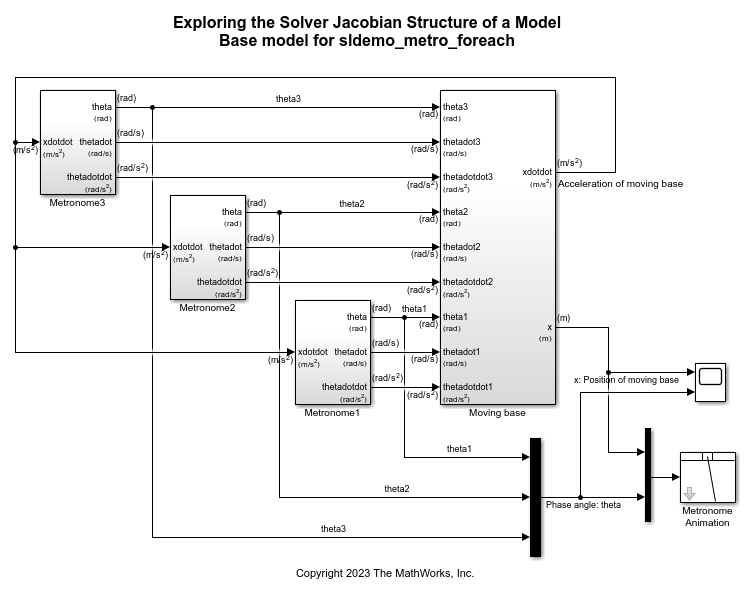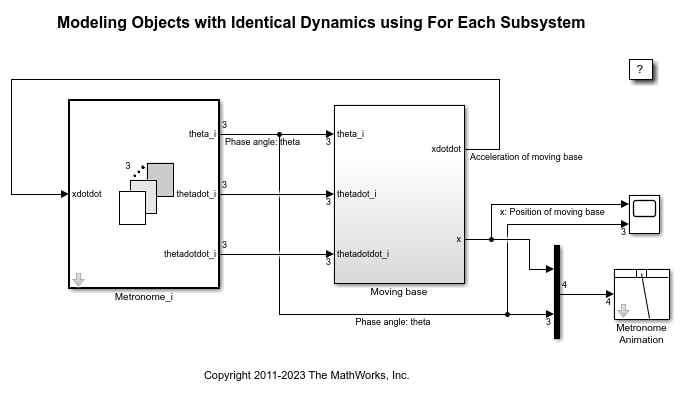# Modeling Objects with Identical Dynamics Using For Each Subsystem

This example shows how to model multiple objects with identical dynamics using the For Each subsystem. The number of objects is parameterized by the length of the input signal.

This example illustrates how you can implement the model `sldemo_metro_basic`, using one For Each subsystem to model three identical metronomes and another to model the identical effect that the motion of each metronome has on the moving base.

The continuous dynamics of the three identical metronomes in sldemo_metro_basic are modeled in identical subsystems: `sldemo_metro_basic/Metronome1`, `sldemo_metro_basic/Metronome2`, and `sldemo_metro_basic/Metronome3`. You can represent these three subsystems as one For Each subsystem. The initial position of the metronomes is passed in as a mask parameter to the For Each subsystem, and is used as an initial condition for one of the integrators. The For Each subsystem is configured to partition this parameter along the first dimension. As a result, an input vector with three elements defines the initial positions for the three metronomes.Figure 1: Metronomes modeled using three subsystems with identical dynamicsFigure 2: Metronomes modeled using one For Each subsystem

On examining the dynamics of the moving base, note that it involves three identical second-order differential terms corresponding to each metronome. You can replace the three copies with one For Each Subsystem. Instead of nine input signals, the For Each subsystem requires only three input signals: the angular displacements, the angular velocities, and the angular accelerations for all metronomes. The For Each subsystem is configured to partition the input signal along the first dimension and operates on one element from each signal for one iteration (i.e. one metronome).Figure 3: Second-order differential terms modeled using copies of the same subsystemFigure 4: Second-order differential terms modeled using one For Each subsystem

After implementing the original model with two For Each subsystems, the model is now parameterized for the number of metronomes. To increase the number of metronomes, introduce a new value to the mask parameter.

For example:

```Theta4 = 0.7568; ```

Double-click on the Metronome_i block to open the mask dialog and change Input Positions (Partitioned) to: [Theta1 Theta2 Theta3 Theta4]

Execute the model to see the displacement angles of the four metronomes and the position of the moving base.

```sim('sldemo_metro_foreach'); ```Figure 5: Simulation animation with For Each subsystem parameterized for four metronomesFigure 6: Simulation result with For Each subsystem parameterized for four metronomes